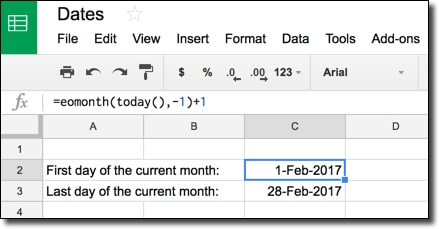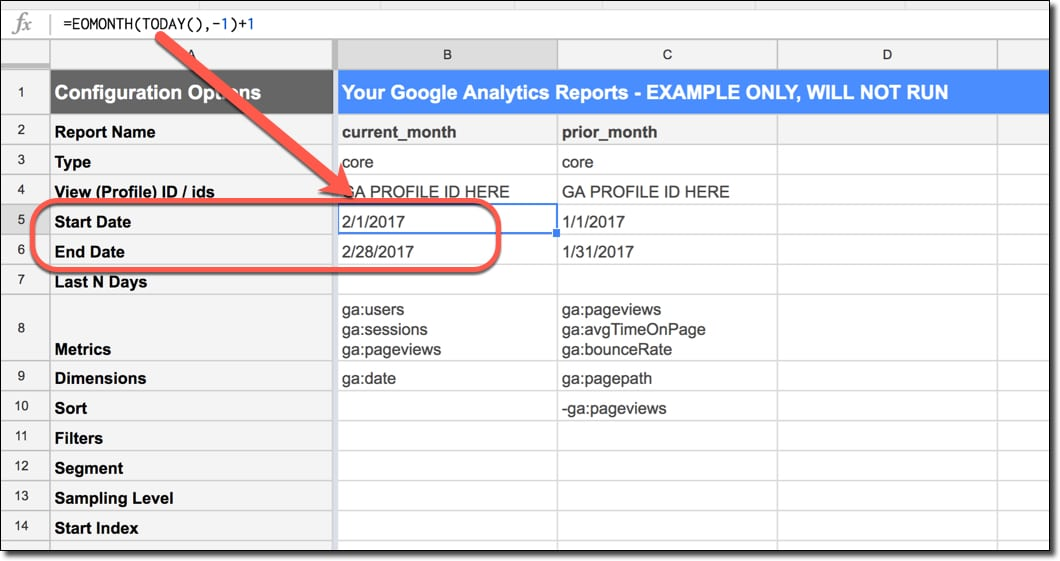# How do I get the first and last days of the current month in Google Sheets?

In this example, we’re going to see how to extract the first and last dates of the current month. You might be using this as a filter on your data to restrict to just looking at your web traffic or revenue in this period.## How do I get the first and last dates of the current month?

We combine two functions, TODAY and EOMONTH, and a little bit of math to create the formulas to extract first and last dates.

## What’s the formula?

For the first day of the current month:

=EOMONTH(TODAY(),-1)+1

For the last day of the current month:

=EOMONTH(TODAY(),0)

## How does this formula work?

The heart of both of these formulas is the function TODAY(), which outputs today’s date in your Sheet. It updates automatically whenever the spreadsheet is recalculated (when you make an edit somewhere else). It’s known as a volatile function because it automatically recalculates, so if you were to have a huge number of these formulas, it would affect the performance of your spreadsheet.

=TODAY()

Next we wrap that with the EOMONTH function to get the date at the end of the month.

For the first day of the current month, we offset by -1 to get the previous month and add 1 day to get the first day of the current month, as follows:

=EOMONTH(TODAY(),-1)+1

The formula for the last day of the current month is simpler. We offset 0, because we want to stay in this same month as the TODAY function, and we don’t need to add a day to the output. So the formula is:

=EOMONTH(TODAY(),0)

These types of date formulas are super useful if you do any data analysis work, and want to group and compare data for set periods. As an example, you may want to automatically generate Start and End Date fields in the Google Analytics Add-On, to always extract the most recent data for current and prior month periods for comparison.See also: We can perform similar calculations to get weekly dates, prior months, quarterly dates and yearly dates.